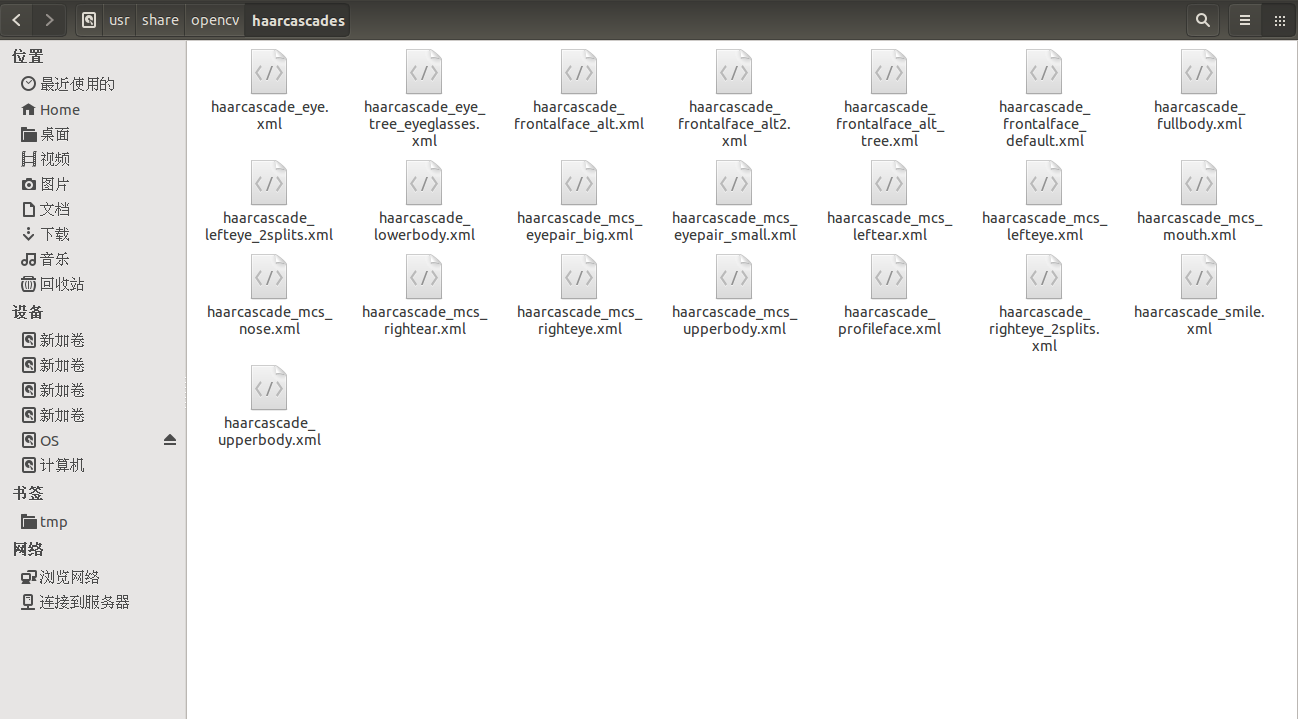# Python-OpenCV人脸检测(代码)

Python-OpenCV人脸检测(代码)

@author:wepon

## 一、软件安装

sudo apt-get install libopencv-*
sudo apt-get install python-opencv
sudo apt-get install python-imaging## 二、python-opencv实现人脸检测

• 人脸检测

def detectFaces(image_name):
if img.ndim == 3:
gray = cv2.cvtColor(img, cv2.COLOR_BGR2GRAY)
else:
gray = img #if语句：如果img维度为3，说明不是灰度图，先转化为灰度图gray，如果不为3，也就是2，原图就是灰度图

faces = face_cascade.detectMultiScale(gray, 1.2, 5)#1.3和5是特征的最小、最大检测窗口，它改变检测结果也会改变
result = []
for (x,y,width,height) in faces:
result.append((x,y,x+width,y+height))
return result

• 截取保存人脸图

def saveFaces(image_name):
faces = detectFaces(image_name)
if faces:
#将人脸保存在save_dir目录下。
#Image模块：Image.open获取图像句柄，crop剪切图像(剪切的区域就是detectFaces返回的坐标)，save保存。
save_dir = image_name.split('.')+"_faces"
os.mkdir(save_dir)
count = 0
for (x1,y1,x2,y2) in faces:
file_name = os.path.join(save_dir,str(count)+".jpg")
Image.open(image_name).crop((x1,y1,x2,y2)).save(file_name)
count+=1

• 框出人脸

#在原图像上画矩形，框出所有人脸。
#调用Image模块的draw方法，Image.open获取图像句柄，ImageDraw.Draw获取该图像的draw实例，然后调用该draw实例的rectangle方法画矩形(矩形的坐标即
#detectFaces返回的坐标)，outline是矩形线条颜色(B,G,R)。
#注：原始图像如果是灰度图，则去掉outline，因为灰度图没有RGB可言。drawEyes、detectSmiles也一样。
def drawFaces(image_name):
faces = detectFaces(image_name)
if faces:
img = Image.open(image_name)
draw_instance = ImageDraw.Draw(img)
for (x1,y1,x2,y2) in faces:
draw_instance.rectangle((x1,y1,x2,y2), outline=(255, 0,0))
img.save('drawfaces_'+image_name)• 眼睛检测

def detectEyes(image_name):
faces = detectFaces(image_name)

gray = cv2.cvtColor(img, cv2.COLOR_BGR2GRAY)
result = []
for (x1,y1,x2,y2) in faces:
roi_gray = gray[y1:y2, x1:x2]
for (ex,ey,ew,eh) in eyes:
result.append((x1+ex,y1+ey,x1+ex+ew,y1+ey+eh))
return result

• 框出眼睛
def drawEyes(image_name):
eyes = detectEyes(image_name)
if eyes:
img = Image.open(image_name)
draw_instance = ImageDraw.Draw(img)
for (x1,y1,x2,y2) in eyes:
draw_instance.rectangle((x1,y1,x2,y2), outline=(0, 0,255))
img.save('draweyes_'+image_name)• 笑脸检测
def detectSmiles(image_name):
if img.ndim == 3:
gray = cv2.cvtColor(img, cv2.COLOR_BGR2GRAY)
else:
gray = img #if语句：如果img维度为3，说明不是灰度图，先转化为灰度图gray，如果不为3，也就是2，原图就是灰度图

result = []
for (x,y,width,height) in smiles:
result.append((x,y,x+width,y+height))
return result


• 框出笑脸
def drawSmiles(image_name):
smiles = detectSmiles(image_name)
if smiles:
img = Image.open(image_name)
draw_instance = ImageDraw.Draw(img)
for (x1,y1,x2,y2) in smiles:
draw_instance.rectangle((x1,y1,x2,y2), outline=(100, 100,0))
img.save('drawsmiles_'+image_name)总之，利用opencv里训练好的haar特征的xml文件，在图片上检测出人脸（眼睛、鼻子、笑脸…）的坐标，利用这个坐标，我们可以将人脸区域剪切保存，也可以在原图上将人脸框出。剪切保存人脸以及用矩形工具框出人脸，本程序使用的是PIL里的Image、ImageDraw模块。此外也可以用opencv里的画图工具画矩形。